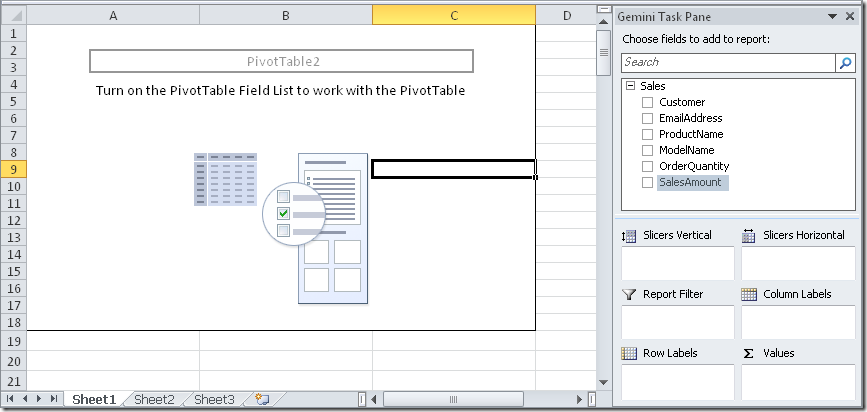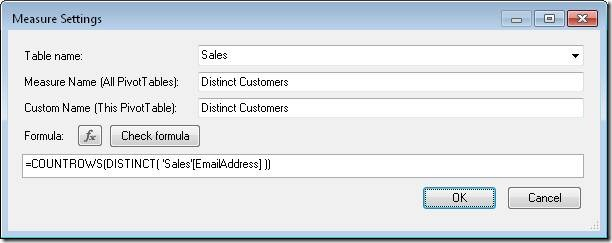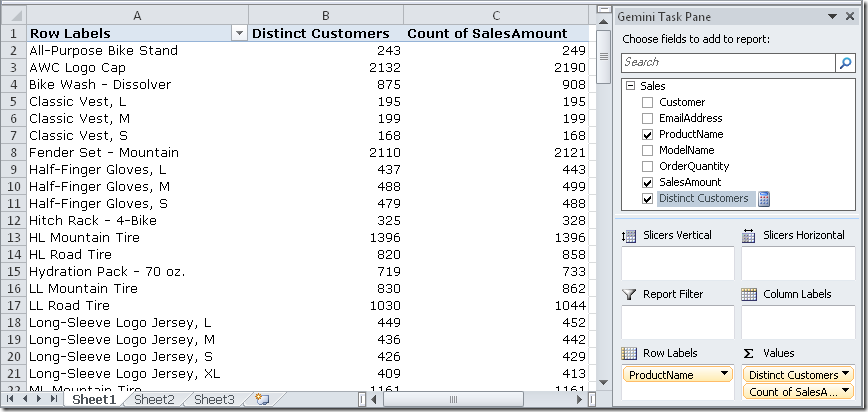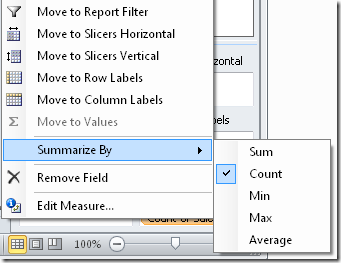UPDATE: PowerPivot for Excel 2010 in SQL Server 2012 and Excel 2013 support the DISTINCTCOUNT aggregation in DAX. This blog post is relevant only if you use PowerPivot for Excel 2010 in SQL Server 2008 R2.

A PivotTable based on PowerPivot data doesn’t offer a native Distinct Count aggregation. To calculate it, you need to create a calculated measure in the PivotTable. For example, if we have a simple Sales table from AdventureWorks loaded in PowerPivot and projected into a PivotTable like the following picture.To create a new measure, we need to right-click on the field pane the menu “Add New Measure…”At this point we name the new measure Distinct Customers and write the DAX formula that calculates the number of rows returned by the DISTINCT function, which returns a list of the distinct values contained in a column (we choose EmailAddress in this example, but if we had a customer key we would have used it instead).The DAX formula is:

COUNTROWS( DISTINCT( Table[Column] ) )

Where Table is the name of the PowerPivot Table containing the column and Column is the name of the column containing the values we want to count as distinct. Having defined this calculated measure, it is computed in the context defined by the PivotTable query. For example, we can look at the number of distinct customers that have bought each product, comparing that number with the number of sales for each product (which is always a number greater than or equal to the number of distinct customers).I’d like to add a personal note. It would have been better if a shorter syntax would have been available, and much better if PivotTable would have offered the Distinct Count as a possible option in the Summarize By options that are available for each native measure (current available options are Sum, Count, Min, Max and Average).Moreover, even a more direct DAX function like a DISTINCTCOUNT( [column] ) would have been easier and intuitive for “that guy”!

DISTINCTCOUNTCounts the number of distinct values in a column.

DISTINCTCOUNT ( <ColumnName> )

DISTINCTReturns a one column table that contains the distinct (unique) values in a column, for a column argument. Or multiple columns with distinct (unique) combination of values, for a table expression argument.

DISTINCT ( <ColumnNameOrTableExpr> )

COUNTROWSCounts the number of rows in a table.

COUNTROWS ( [<Table>] )